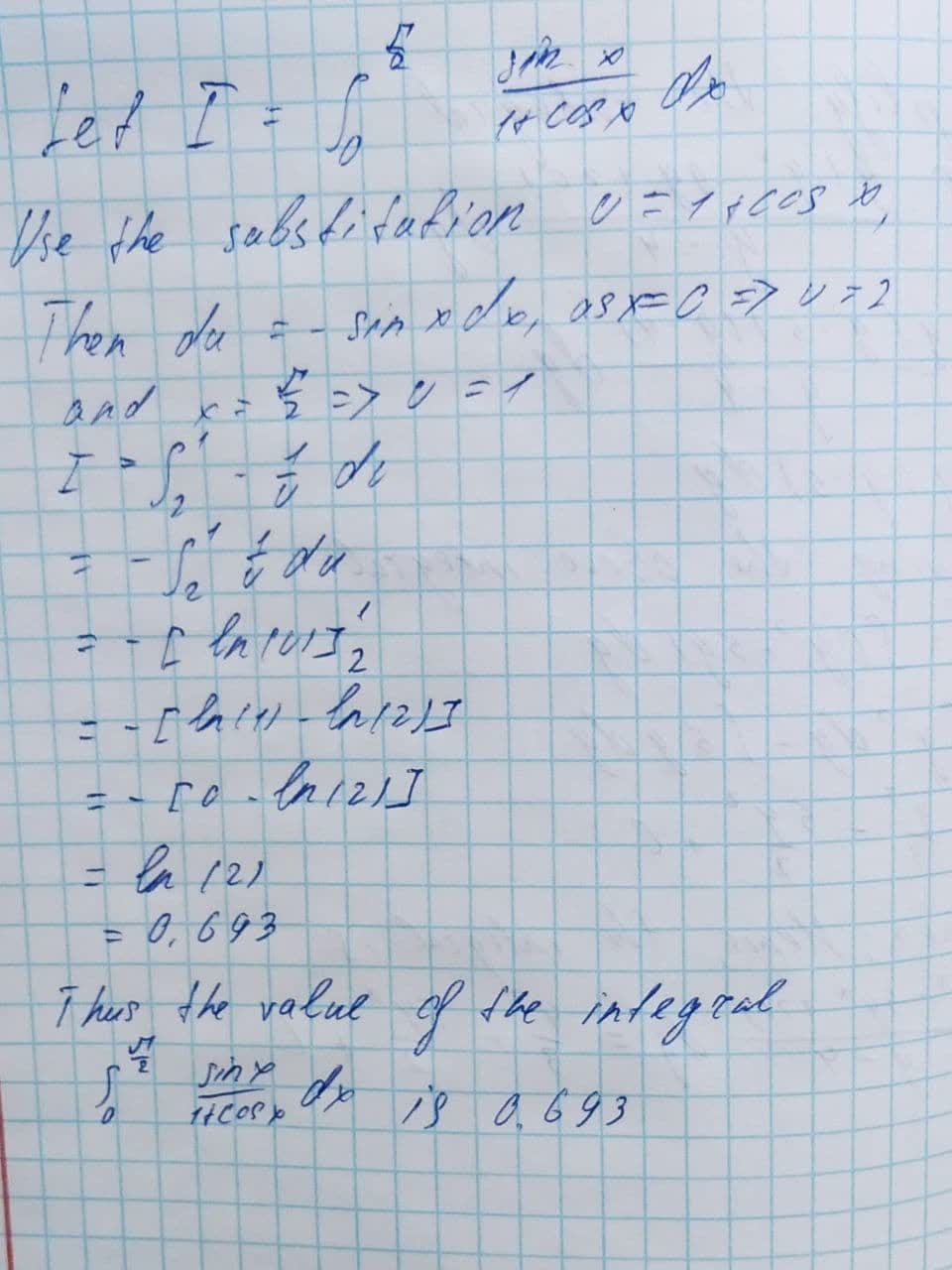Question# Evaluate the following integrals and include absolute values: \int_{0}^{\frac{\pi}{2}}\frac{\sin x}{1+\cos x}dx

Applications of integrals
ANSWEREDEvaluate the following integrals. Include absolute values only when needed.
$$\displaystyle{\int_{{{0}}}^{{{\frac{{\pi}}{{{2}}}}}}}{\frac{{{\sin{{x}}}}}{{{1}+{\cos{{x}}}}}}{\left.{d}{x}\right.}$$